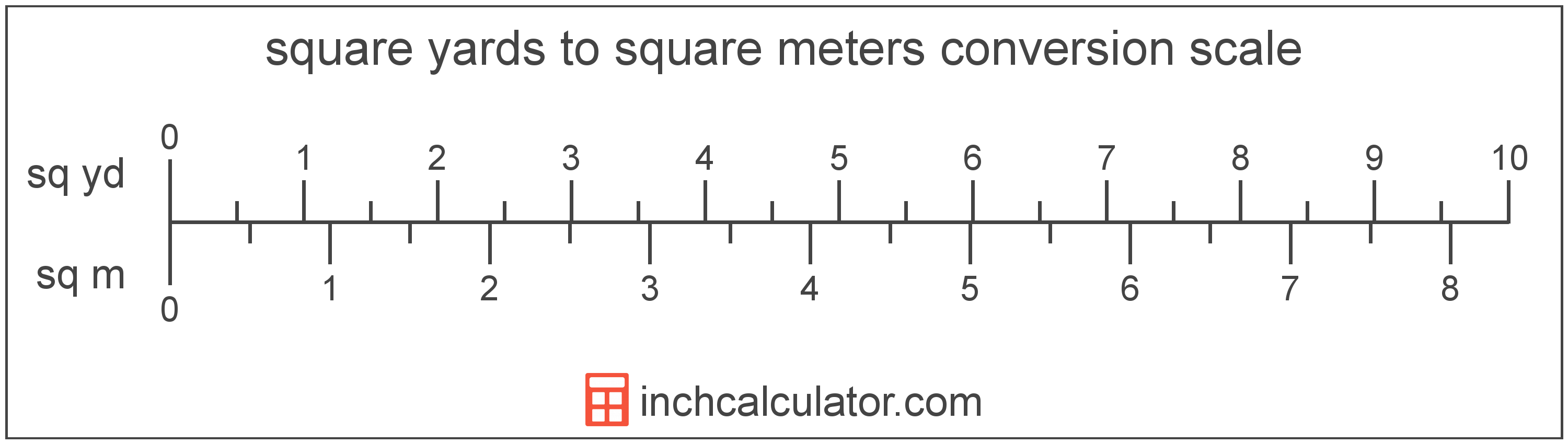# Square Meters to Square Yards Converter

Enter the area in square meters below to get the value converted to square yards.

(find square meters)
Results in Square Yards:1 sq m = 1.19599 sq yd

Do you want to convert square yards to square meters?

## How to Convert Square Meters to Square Yards

To convert a measurement in square meters to a measurement in square yards, multiply the area by the following conversion ratio: 1.19599 square yards/square meter.

Since one square meter is equal to 1.19599 square yards, you can use this simple formula to convert:

square yards = square meters × 1.19599

The area in square yards is equal to the area in square meters multiplied by 1.19599.

For example, here's how to convert 5 square meters to square yards using the formula above.
square yards = (5 sq m × 1.19599) = 5.97995 sq yd### How Many Square Yards Are in a Square Meter?

There are 1.19599 square yards in a square meter, which is why we use this value in the formula above.

1 sq m = 1.19599 sq yd

## What is a Square Meter?

One square meter is equivalent to the area of a square with sides that are each 1 meter in length. One square meter is equal to 10.76391 square feet or 10,000 square centimeters.

The square meter, or square metre, is the SI derived unit for area in the metric system. A square meter is sometimes also referred to as a square m. Square meters can be abbreviated as sq m, and are also sometimes abbreviated as . For example, 1 square meter can be written as 1 sq m or 1 m².

You can use a square meters calculator to calculate the area of a space if you know its dimensions.

## What is a Square Yard?

One square yard is equivalent to the area of a square with sides that are each 1 yard in length. One square yard is roughly equal to 9 square feet or 0.836127 square meters.

The square yard is a US customary and imperial unit of area. A square yard is sometimes also referred to as a square yd. Square yards can be abbreviated as sq yd, and are also sometimes abbreviated as yd². For example, 1 square yard can be written as 1 sq yd or 1 yd².

You can use a square yards calculator to calculate the area of a space if you know its dimensions.

## Square Meter to Square Yard Conversion Table

Table showing various square meter measurements converted to square yards.
Square Meters Square Yards
1 sq m 1.196 sq yd
2 sq m 2.392 sq yd
3 sq m 3.588 sq yd
4 sq m 4.784 sq yd
5 sq m 5.98 sq yd
6 sq m 7.1759 sq yd
7 sq m 8.3719 sq yd
8 sq m 9.5679 sq yd
9 sq m 10.76 sq yd
10 sq m 11.96 sq yd
11 sq m 13.16 sq yd
12 sq m 14.35 sq yd
13 sq m 15.55 sq yd
14 sq m 16.74 sq yd
15 sq m 17.94 sq yd
16 sq m 19.14 sq yd
17 sq m 20.33 sq yd
18 sq m 21.53 sq yd
19 sq m 22.72 sq yd
20 sq m 23.92 sq yd
21 sq m 25.12 sq yd
22 sq m 26.31 sq yd
23 sq m 27.51 sq yd
24 sq m 28.7 sq yd
25 sq m 29.9 sq yd
26 sq m 31.1 sq yd
27 sq m 32.29 sq yd
28 sq m 33.49 sq yd
29 sq m 34.68 sq yd
30 sq m 35.88 sq yd
31 sq m 37.08 sq yd
32 sq m 38.27 sq yd
33 sq m 39.47 sq yd
34 sq m 40.66 sq yd
35 sq m 41.86 sq yd
36 sq m 43.06 sq yd
37 sq m 44.25 sq yd
38 sq m 45.45 sq yd
39 sq m 46.64 sq yd
40 sq m 47.84 sq yd

## References

1. Collins Dictionary, Definition of 'square meter', https://www.collinsdictionary.com/us/dictionary/english/square-meter